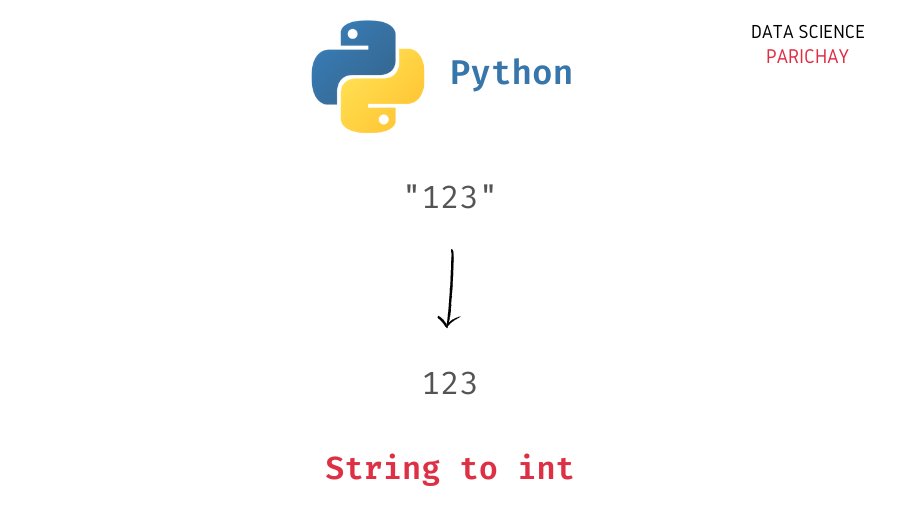# Convert String to Integer in Python

In this tutorial, we will look at how to convert a string in Python to an integer, `int` datatype with the help of some examples.

## How to convert string to int in Python?

You can use the Python built-in `int()` function to convert a string to an integer in Python. The following is the syntax –

```# convert string s to int
int(s)```

It returns the passed string as an integer. Note that if the passed string does not represent a whole number, it will raise an error (even with float numbers represented as string).

## Examples

Let’s look at some examples of using the above syntax to convert a string to an integer.

First, let’s apply the `int()` function on a string containing a whole number, for example, “150”.

```# string storing a number
s = "150"
# convert string to int
num = int(s)
# display integer and its type
print(num)
print(type(num))```

Output:

```150
<class 'int'>```

You can see that we get `150` as an integer.

What happens if you pass a floating-point number as a string to the `int()` function? Let’s find out.

📚 Data Science Programs By Skill Level

Introductory

Intermediate ⭐⭐⭐

🔎 Find Data Science Programs 👨‍💻 111,889 already enrolled

Disclaimer: Data Science Parichay is reader supported. When you purchase a course through a link on this site, we may earn a small commission at no additional cost to you. Earned commissions help support this website and its team of writers.

```# string storing a floating point number
s = "150.75"
# convert string to int
num = int(s)
# display integer and its type
print(num)
print(type(num))```

Output:

```---------------------------------------------------------------------------
ValueError                                Traceback (most recent call last)
Input In , in <module>
2 s = "150.75"
3 # convert string to int
----> 4 num = int(s)
5 # display integer and its type
6 print(num)

ValueError: invalid literal for int() with base 10: '150.75'```

Here we pass “150.75” to the `int()` function. Since the string passed is not a whole number, we get an error. If you want to convert the above string to a numeric form in Python, use the `float()` function instead.

```# string storing a floating point number
s = "150.75"
# convert string to float
num = float(s)
# display number and its type
print(num)
print(type(num))```

Output:

```150.75
<class 'float'>```

We get the string as a float value.

What happens if you pass a non-numeric string to the `int()` function? Let’s find out.

```# string with text
s = "cat"
# convert string to int
num = int(s)
# display number and its type
print(num)
print(type(num))```

Output:

```---------------------------------------------------------------------------
ValueError                                Traceback (most recent call last)
Input In , in <module>
2 s = "cat"
3 # convert string to int
----> 4 num = int(s)
5 # display number and its type
6 print(num)

ValueError: invalid literal for int() with base 10: 'cat'```

As expected, we get an error because the passed string cannot be represented as an integer.

You might also be interested in –

•